## 3 Functional description of the RPE‑LTP codec

06.103GPPFull Rate Speech TranscodingTS

The block diagram of the RPE‑LTP‑coder is shown in figure 3.1. The individual blocks are described in the following subclauses.

## 3.1 Functional description of the RPE‑LTP encoder

The Pre‑processing section of the RPE‑LTP encoder comprises the following two sub‑blocks:

‑ Offset compensation (3.1.1);

‑ Pre‑emphasis (3.1.2).

The LPC analysis section of the RPE‑LTP encoder comprises the following five sub‑blocks:

‑ Segmentation (3.1.3);

‑ Auto‑Correlation (3.1.4);

‑ Schur Recursion (3.1.5);

‑ Transformation of reflection coefficients to Log.‑Area Ratios (3.1.6);

‑ Quantization and coding of Log.‑Area Ratios (3.1.7).

The Short term analysis filtering section of the RPE‑LTP comprises the following four sub‑blocks:

‑ Decoding of the quantized Log.‑Area Ratios (LARs) (3.1.8);

‑ Interpolation of Log.‑Area Ratios (3.1.9);

‑ Transformation of Log.‑Area Ratios into reflection coefficients (3.1.10);

‑ Short term analysis filtering (3.1.11).

The Long Term Predictor (LTP) section comprises 4 sub‑blocks working on subsegments (3.1.12) of the short term residual samples:

‑ Calculation of LTP parameters (3.1.13);

‑ Coding of the LTP lags (3.1.14) and the LTP gains (3.1.15);

‑ Decoding of the LTP lags (3.1.14) and the LTP gains (3.1.15);

‑ Long term analysis filtering (3.1.16), and Long term synthesis filtering (3.1.17).

The RPE encoding section comprises five different sub‑blocks:

‑ Weighting filter (3.1.18);

‑ Adaptive sample rate decimation by RPE grid selection (3.1.19);

‑ APCM quantization of the selected RPE sequence (3.1.20);

‑ APCM inverse quantization (3.1.21);

‑ RPE grid positioning (3.1.22).

Pre‑processing section

### 3.1.1 Offset compensation

Prior to the speech encoder an offset compensation, by a notch filter is applied in order to remove the offset of the input signal so to produce the offset‑free signal sof.

sof(k) = so(k) – so(k‑1) + alpha*sof(k‑1) (3.1.1)

alpha = 32735*2‑15

### 3.1.2 Pre‑emphasis

The signal sof is applied to a first order FIR pre‑emphasis filter leading to the input signal s of the analysis section.

s(k) = sof(k) – beta*sof(k‑1) (3.1.2)

beta= 28180*2‑15

LPC analysis section

### 3.1.3 Segmentation

The speech signal s(k) is divided into non‑overlapping frames having a length of T0 = 20 ms (160 samples). A new LPC‑analysis of order p=8 is performed for each frame.

### 3.1.4 Autocorrelation

The first p+1 = 9 values of the Auto‑Correlation function are calculated by:

159

ACF(k)=  s(i)s(i-k) ,k = 0,1…,8 (3.2)

i=k

### 3.1.5 Schur Recursion

The reflection coefficients are calculated as shown in figure 3.2 using the Schur Recursion algorithm. The term "reflection coefficient" comes from the theory of linear prediction of speech (LPC), where a vocal tract representation consisting of series of uniform cylindrical sections is assumed. Such a representation can be described by the reflection coefficients or the area ratios of connected sections.

### 3.1.6 Transformation of reflection coefficients to Log.‑Area Ratios

The reflection coefficients r(i), (i=1..8), calculated by the Schur algorithm, are in the range:

‑1 <= r(i) <= + 1

Due to the favourable quantization characteristics, the reflection coefficients are converted into Log.‑Area Ratios which are strictly defined as follows:

1 + r(i)

Logarea(i) = log10 (———-) (3.3)

1 – r(i)

Since it is the companding characteristic of this transformation that is of importance, the following segmented approximation is used.

r(i) ; |r(i)| < 0.675

LAR(i) = sign[r(i)]*[2|r(i)|‑0.675] ; 0.675 <= |r(i)| < 0.950

sign[r(i)]*[8|r(i)|‑6.375] ; 0.950 <= |r(i)| <= 1.000

(3.4)

with the result that instead of having to divide and obtain the logarithm of particular values, it is merely necessary to multiply, add and compare these values.

The following equation (3.5) gives the inverse transformation.

LAR'(i) ; |LAR'(i)|<0.675

r'(i)=sign[LAR'(i)]*[0.500*|LAR'(i)|

+0.337500] ; 0.675<=|LAR'(i)|<1.225

sign[LAR'(i)]*[0.125*|LAR'(i)|

+0.796875] ; 1.225<=|LAR'(i)|<=1.625

(3.5)

### 3.1.7 Quantization and coding of Log.‑Area Ratios

The Log.‑Area Ratios LAR(i) have different dynamic ranges and different asymmetric distribution densities. For this reason, the transformed coefficients LAR(i) are limited and quantized differently according to the following equation (3.6), with LARc(i) denoting the quantized and integer coded version of LAR(i).

LARc(i) = Nint{A(i)*LAR(i) + B(i)} (3.6)

with

Nint{z} = int{z+sign{z}*0.5} (3.6a)

Function Nint defines the rounding to the nearest integer value, with the coefficients A(i), B(i), and different extreme values of LARc(i) for each coefficient LAR(i) given in table 3.1.

Table 3.1: Quantization of the Log.‑Area Ratios LAR(i)

 LAR No i A(i) B(i) Minimum LARc(i) Maximum LARc(i) 1 20.000 0.000 ‑32 +31 2 20.000 0.000 ‑32 +31 3 20.000 4.000 ‑16 +15 4 20.000 ‑5.000 ‑16 +15 5 13.637 0.184 ‑ 8 + 7 6 15.000 ‑3.500 ‑ 8 + 7 7 8.334 ‑0.666 ‑ 4 + 3 8 8.824 ‑2.235 ‑ 4 + 3

Short‑term analysis filtering section

The current frame of the speech signal s is retained in memory until calculation of the LPC parameters LAR(i) is completed. The frame is then read out and fed to the short term analysis filter of order p=8. However, prior to the analysis filtering operation, the filter coefficients are decoded and pre‑processed by interpolation.

### 3.1.8 Decoding of the quantized Log.‑Area Ratios

In this block the quantized and coded Log.‑Area Ratios (LARc(i)) are decoded according to equation (3.7).

LAR”(i) = ( LARc(i) – B(i) )/ A(i) (3.7)

### 3.1.9 Interpolation of Log.‑Area Ratios

To avoid spurious transients which may occur if the filter coefficients are changed abruptly, two subsequent sets of Log.‑Area Ratios are interpolated linearly. Within each frame of 160 analysed speech samples the short term analysis filter and the short term synthesis filter operate with four different sets of coefficients derived according to table 3.2.

Table 3.2: Interpolation of LAR parameters (J=actual segment)

 k LAR’J(i) = 0…12 0.75*LAR’ ‘J‑1(i) + 0.25*LAR’ ‘J(i) 13…26 0.50*LAR’ ‘J‑1(i) + 0.50*LAR’ ‘J(i) 27…39 0.25*LAR’ ‘J‑1(i) + 0.75*LAR’ ‘J(i) 40..159 LAR’ ‘J(i)

### 3.1.10 Transformation of Log.‑Area Ratios into reflection coefficients

The reflection coefficients are finally determined using the inverse transformation according to equation (3.5).

### 3.1.11 Short term analysis filtering

The Short term analysis filter is implemented according to the lattice structure depicted in figure 3.3.

d0(k) = s(k) (3.8a)

u0(k) = s(k) (3.8b)

di(k) = di‑1(k) + r’i*ui‑1(k‑1) with i=1,…8 (3.8c)

ui(k) = ui‑1(k‑1) + r’i*di‑1(k) with i=1,…8 (3.8d)

d(k ) = d8(k) (3.8e)

Long‑Term Predictor (LTP) section

### 3.1.12 Sub‑segmentation

Each input frame of the short term residual signal contains 160 samples, corresponding to 20 ms. The long term correlation is evaluated four times per frame, for each 5 ms subsegment. For convenience in the following, we note j=0,…,3 the sub‑segment number, so that the samples pertaining to the j‑th sub‑segment of the residual signal are now denoted by d(kj+k) with j = 0,…,3; kj = k0 + j*40 and k = 0,…,39 where k0 corresponds to the first value of the current frame.

### 3.1.13 Calculation of the LTP parameters

For each of the four sub‑segments a long term correlation lag Nj, (j=0,…,3), and an associated gain factor bj, (j=0,…,3) are determined. For each sub‑segment, the determination of these parameters is implemented in three steps.

1) The first step is the evaluation of the cross‑correlation Rj(lambda) of the current sub‑segment of short term residual signal d(kj+i),(i=0,…,39) and the previous samples of the reconstructed short term residual signal d'(kj+i), (i=‑120,…,‑1):

39 j = 0,…3

Rj(lambda) =  d(kj+i)*d'(kj+i-lambda); kj = k0 + j*40

i=0 lambda = 40,…,120

(3.9)

The cross‑correlation is evaluated for lags lambda greater than or equal to 40 and less than or equal to 120, i.e. corresponding to samples outside the current sub‑segment and not delayed by more than two sub‑segments.

2) The second step is to find the position Nj of the peak of the cross‑correlation function within this interval:

Rj(Nj) = max { Rj(lambda); lambda = 40..120 };

j = 0,…,3

(3.10)

3) The third step is the evaluation of the gain factor bj according to:

bj = Rj(Nj) / Sj(Nj); j = 0,…,3 (3.11)

with

39

Sj(Nj) =  d’2 (kj+i-Nj); j = 0,…,3 (3.12)

i=0

It is clear that the last 120 samples of the reconstructed short term residual signal d'(kj+i),(i=‑120,…,‑1) shall be retained until the next sub‑segment so as to allow the evaluation of the relations (3.9),…,(3.12).

### 3.1.14 Coding/Decoding of the LTP lags

The long term correlation lags Nj,(j=0,…,3) can have values in the range (40,…,120), and so shall be coded using 7 bits with:

Ncj = Nj; j = 0,…,3 (3.13)

At the receiving end, assuming an error free transmission, the decoding of these values will restore the actual lags:

Nj’ = Ncj; j = 0,…,3 (3.14)

### 3.1.15 Coding/Decoding of the LTP gains

The long term prediction gains bj,(j=0,…,3) are encoded with 2 bits each, according to the following algorithm:

if bj <= DLB(i) then bcj = 0; i=0

if DLB(i‑1) < bj <= DLB(i) then bcj = i; i=1,2 (3.15)

if DLB(i‑1) < bj then bcj = 3; i=3

where DLB(i),(i=0,…,2) denotes the decision levels of the quantizer, and bcj represents the coded gain value. Decision levels and quantizing levels are given in table 3.3.

Table 3.3: Quantization table for the LTP gain

 i Decision level Quantizing level DLB(i) QLB(i) 0 0.2 0.10 1 0.5 0.35 2 0.8 0.65 3 1.00

The decoding rule is implemented according to:

bj’ = QLB(bcj) ; j = 0,…,3 (3.16)

where QLB(i),(i=0,…,3) denotes the quantizing levels, and bj’ represents the decoded gain value (see table 3.3).

### 3.1.16 Long term analysis filtering

The short term residual signal d(k0+k),(k=0,…,159) is processed by sub‑segments of 40 samples. From each of the four sub‑segments (j=0,…,3) of short term residual samples, denoted here d(kj+k), (k=0,…,39), an estimate d"(kj+k), (k=0,…,39) of the signal is subtracted to give the long term residual signal e(kj+k), (k=0,…,39) (see figure 3.1):

j = 0,…,3

e(kj+k) = d(kj+k) – d"(kj+k) ; k = 0,…,39 (3.17)

kj = k0 + j*40

Prior to this subtraction, the estimated samples d"(kj+k) are computed from the previously reconstructed short term residual samples d’, adjusted to the current sub‑segment LTP lag Nj’ and weighted with the sub‑segment LTP gain bj’:

j = 0,…,3

d"(kj+k) = bj’*d'(kj+k-Nj’) ; k = 0,…,39 (3.18)

kj = k0 + j*40

### 3.1.17 Long term synthesis filtering

The reconstructed long term residual signal e'(k0+k),(k=0,…,159) is processed by sub‑segments of 40 samples. To each sub‑segment, denoted here e'(kj+k), (k=0,…,39), the estimate d"(kj+k), (k=0,…,39) of the signal is added to give the reconstructed short term residual signal d'(kj+k),(k=0,…,39):

j = 0,…,3

d'(kj+k) = e'(kj+k) + d"(kj+k) ; k = 0,…,39 (3.19)

kj = k0 + j*40

RPE encoding section

### 3.1.18 Weighting Filter

A FIR "block filter" algorithm is applied to each sub‑segment by convolving 40 samples e(k) with the impulse response H(i) ; i=0,…,10 (see table 3.4).

Table 3.4: Impulse response of block filter (weighting filter)

 i 5 4 (6) 3 (7) 2 (8) 1 (9) 0 (10) H(i)*213 8192 5741 2054 0 ‑374 ‑134

|H(Omega=0)| = 2.779;

The conventional convolution of a sequence having 40 samples with an 11‑tap impulse response would produce 40+11‑1=50 samples. In contrast to this, the "block filter" algorithm produces the 40 central samples of the conventional convolution operation. For notational convenience the block filtered version of each sub‑segment is denoted by x(k), k=0,…,39.

10

x(k) =  H(i) * e(k+5-i) with k = 0,…,39 (3.20)

i=0

NOTE: e(k+5‑i) = 0 for k+5‑i<0 and k+5‑i>39.

### 3.1.19 Adaptive sample rate decimation by RPE grid selection

For the next step, the filtered signal x is down‑sampled by a ratio of 3 resulting in 3 interleaved sequences of lengths 14, 13 and 13, which are split up again into 4 sub‑sequences xm of length 13:

xm(i) = x(kj+m+3*i) ; i = 0,…,12 (3.21)

m = 0,…,3

with m denoting the position of the decimation grid. According to the explicit solution of the RPE mean squared error criterion, the optimum candidate sub‑sequence xM is selected which is the one with the maximum energy:

12

EM = max  xm2(i) ; m = 0,…,3 (3.22)

m i=0

The optimum grid position M is coded as Mc with 2 bits.

### 3.1.20 APCM quantization of the selected RPE sequence

The selected sub‑sequence xM(i) (RPE sequence) is quantized, applying APCM (Adaptive Pulse Code Modulation). For each RPE sequence consisting of a set of 13 samples xM(i) ,the maximum xmax of the absolute values |xM(i)| is selected and quantized logarithmically with 6 bits as xmaxc as given in table 3.5.

Table 3.5: Quantization of the block maximum xmax

 xmax x’max xmaxc xmax x’max xmaxc 0 .. 31 31 0 2048 .. 2303 2303 32 32 .. 63 63 1 2304 .. 2559 2559 33 64 .. 95 95 2 2560 .. 2815 2815 34 96 .. 127 127 3 2816 .. 3071 3071 35 128 .. 159 159 4 3072 .. 3327 3327 36 160 .. 191 191 5 3328 .. 3583 3583 37 192 .. 223 223 6 3584 .. 3839 3839 38 224 .. 255 255 7 3840 .. 4095 4095 39 256 .. 287 287 8 4096 .. 4607 4607 40 288 .. 319 319 9 4608 .. 5119 5119 41 320 .. 351 351 10 5120 .. 5631 5631 42 352 .. 383 383 11 5632 .. 6143 6143 43 384 .. 415 415 12 6144 .. 6655 6655 44 416 .. 447 447 13 6656 .. 7167 7167 45 448 .. 479 479 14 7168 .. 7679 7679 46 480 .. 511 511 15 7680 .. 8191 8191 47 512 .. 575 575 16 8192 .. 9215 9215 48 576 .. 639 639 17 9216 .. 10239 10239 49 640 .. 703 703 18 10240 .. 11263 11263 50 704 .. 767 767 19 11264 .. 12287 12287 51 768 .. 831 831 20 12288 .. 13311 13311 52 832 .. 895 895 21 13312 .. 14335 14335 53 896 .. 959 959 22 14336 .. 15359 15359 54 960 .. 1023 1023 23 15360 .. 16383 16383 55 1024 .. 1151 1151 24 16384 .. 18431 18431 56 1152 .. 1279 1279 25 18432 .. 20479 20479 57 1280 .. 1407 1407 26 20480 .. 22527 22527 58 1408 .. 1535 1535 27 22528 .. 24575 24575 59 1536 .. 1663 1663 28 24576 .. 26623 26623 60 1664 .. 1791 1791 29 26624 .. 28671 28671 61 1792 .. 1919 1919 30 28672 .. 30719 30719 62 1920 .. 2047 2047 31 30720 .. 32767 32767 63

For the normalization, the 13 samples are divided by the decoded version x’max of the block maximum. Finally, the normalized samples:

x'(i) = xM(i)/x’max ; i=0,…,12 (3.23)

are quantized uniformly with three bits to xMc(i) as given in table 3.6.

Table 3.6: Quantization of the normalized RPE‑samples

 x’*215 xM’*215 xMc (Interval‑limits) (Channel) ‑32768 … ‑24577 ‑28672 0 = 000 ‑24576 … ‑16385 ‑20480 1 = 001 ‑16384 … ‑8193 ‑12288 2 = 010 ‑8192 … ‑1 ‑4096 3 = 011 0 … 8191 4096 4 = 100 8192 … 16383 12288 5 = 101 16384 … 24575 20480 6 = 110 24576 … 32767 28672 7 = 111

### 3.1.21 APCM inverse quantization

The xMc(i) are decoded to xM'(i) and denormalized using the decoded value x’maxc leading to the decoded sub‑sequence x’M(i).

### 3.1.22 RPE grid positioning

The quantized sub‑sequence is upsampled by a ratio of 3 by inserting zero values according to the grid position given with Mc.

## 3.2 Decoder

The decoder comprises the following 4 sections. Most of the sub‑blocks are also needed in the encoder and have been described already. Only the short term synthesis filter and the de‑emphasis filter are added in the decoder as new sub‑blocks.

‑ RPE decoding section (3.2.1);

‑ Long Term Prediction section (3.2.2);

‑ Short term synthesis filtering section (3.2.3);

‑ Post‑processing (3.2.4).

The complete block diagram for the decoder is shown in figure 3.4. The variables and parameters of the decoder are marked by the index r to distinguish the received values from the encoder values.

### 3.2.1 RPE decoding section

The input signal of the long term synthesis filter (reconstruction of the long term residual signal) is formed by decoding and denormalizing the RPE‑samples (APCM inverse quantization ‑ 3.1.21) and by placing them in the correct time position (RPE grid positioning ‑ 3.1.22). At this stage, the sampling frequency is increased by a factor of 3 by inserting the appropriate number of intermediate zero‑valued samples.

### 3.2.2 Long Term Prediction section

The reconstructed long term residual signal er’ is applied to the long term synthesis filter (see 3.1.16 and 3.1.17) which produces the reconstructed short term residual signal dr’ for the short term synthesizer.

### 3.2.3 Short term synthesis filtering section

The coefficients of the short term synthesis filter (see figure 3.5) are reconstructed applying the identical procedure to that in the encoder (3.1.8 ‑ 3.1.10). The short term synthesis filter is implemented according to the lattice structure depicted in figure 3.5.

sr(0)(k) = dr'(k) (3.24a)

sr(i)(k) = sr(i‑1)(k) – rr'(9-i) * v8-i(k‑1); i=1,…,8

(3.24b)

v9-i(k) = v8-i(k‑1) + rr'(9-i) * sr(i)(k); i=1,…,8

(3.24c)

sr'(k) = sr(8)(k) (3.24d)

v0(k) = sr(8)(k) (3.24e)

### 3.2.4 Post‑processing

The output of the synthesis filter sr(k) is fed into the IIR‑ de‑emphasis filter leading to the output signal sro.

sro(k) = sr(k) + beta*sro(k‑1) ; beta= 28180*2‑15 (3.25)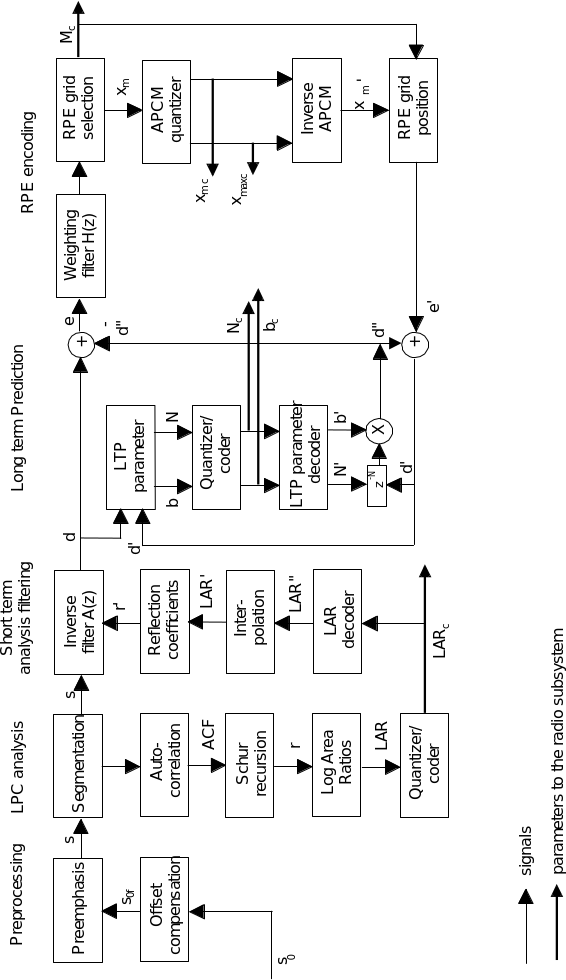Figure 3.1: Block diagram of the RPE ‑ LTP encoder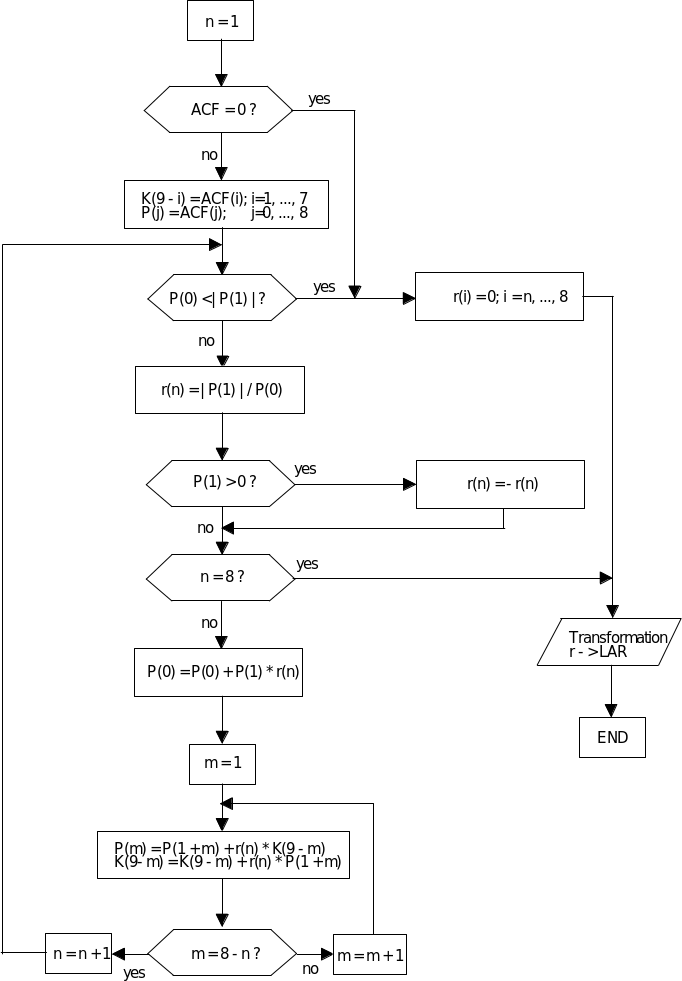Figure 3.2: LPC analysis using Schur recursion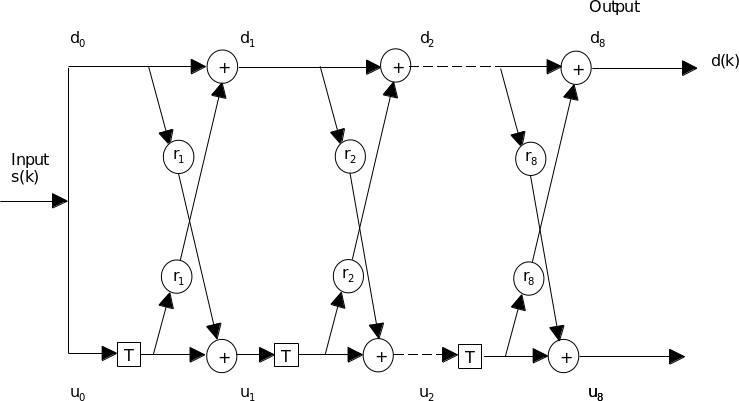Figure 3.3: Short term analysis filter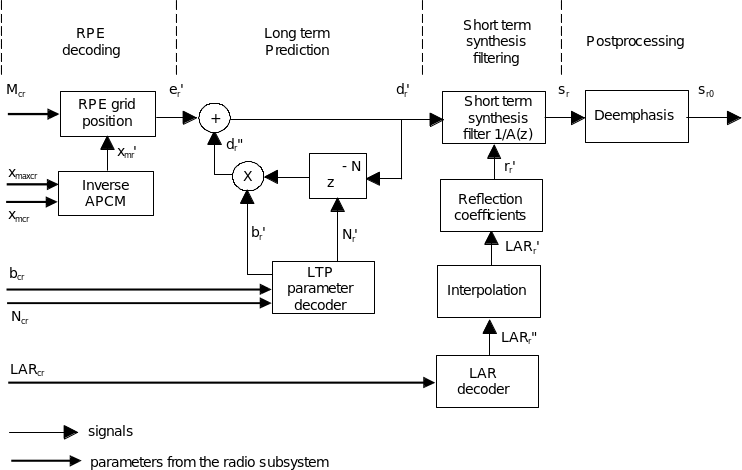Figure 3.4: Block diagram of the RPE‑LTP decoder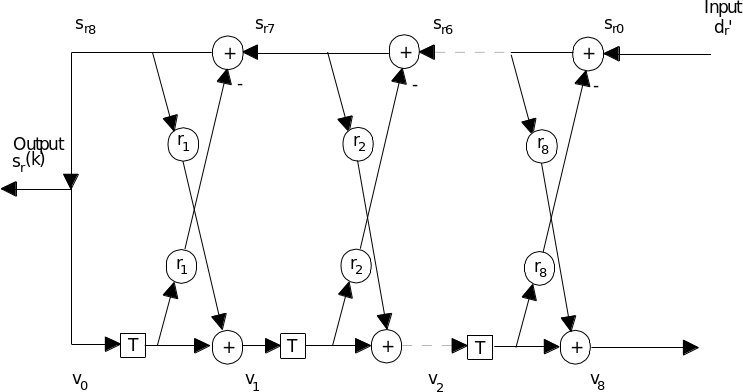Figure 3.5: Short term synthesis filter